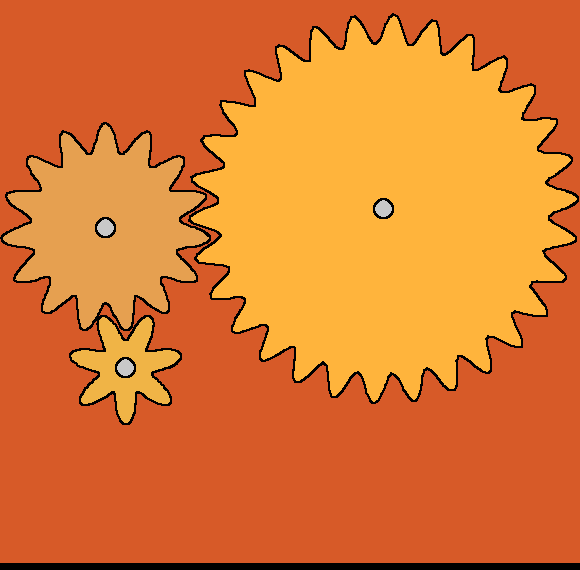# Gear drawing- and animationThis started with wondering how easy it is to draw convincing gear teeth- although the shape I made is not the correct smooth-meshing profile. Then I moved on to animating meshing gears, cheating by saving successive stills then making the animaed GIF.

OK got meshing working... if a little flashy when running.```    nomainwin

global hdc, pi

WindowWidth  =600
WindowHeight =600

open "test" for graphics_nsb_nf as #gr

#gr "trapclose [quit]"
#gr "down"

targetcolor     =0  '   this is the colour of the outline to fill out to.
c               =0

hw      =hwnd( #gr)    '                                    <<<<<<<<<<<<<<<<<<<<<<<<<<<<<<<<<<<<<<
calldll #user32, "GetDC", hw as ulong, hdc as ulong    '    <<<<<<<<<<<<<<<<<<<<<<<<<<<<<<<<<<<<<<

pi      =acs( -1)

#gr "cls ; down ; fill 180 180 60 ; up ; size 2"

phase =0

do

#gr "cls ; down ; fill 180 180 180 ; up ; color 0 0 0"

call gear 385, 210, 30, "255 180 60", phase, "CCW"
call gear 107, 229, 15, "230 160 80", phase, "CW"

#gr "flush"

#gr "getbmp drawing 1 1 580 570"
bmpsave "drawing", "meshing" +right\$( "000" +str\$( c), 3) +".bmp"
c       =c +1

scan

calldll #kernel32, "Sleep", 200 as long, ret as void

phase   =phase +pi /10

loop until phase >2 *pi

wait

sub gear xPos, yPos, numTeeth, phillCol\$, phase, sense\$
ratio       =1'30 /numTeeth

r       =calcdRadius +10 *sin( numTeeth *phase)
#gr "up ; goto "; xPos +r *cos( phase); " "; yPos +r *sin( phase)

for phi =0 to 2 *pi +0.1 step 0.02
r   =calcdRadius +15 *sin( numTeeth *phi +phase *ratio)

if sense\$ ="CW" then
x   =xPos +r *cos( phi)
y   =yPos +r *sin( phi)
else
x   =xPos +r *cos( phi)
y   =yPos -r *sin( phi)
end if

#gr "goto "; int( x); " "; int( y)
#gr "down"
'calldll #kernel32, "Sleep", 2 as long, ret as void

next phi

#gr "backcolor 200 200 200"
#gr "up ; goto "; xPos; " "; yPos; " ; down ; circlefilled 10"
xVar =xPos +20
yVar =yPos

#gr "backcolor "; phillCol\$

calldll #gdi32, "ExtFloodFill",_
hdc                as ulong,_
xVar               as long,_
yVar               as long,_
targetcolor        as long,_
_FLOODFILLBORDER   as long,_    '       '   ie fill out 'til targetcolour is met
result             as long

#gr "up"
end sub

[quit]
close #gr
calldll #user32, "ReleaseDC", hw as ulong, hdc as ulong, ret as void   'release the DC    <<<<<<<<<<<<<<<<<<<<<
end
```